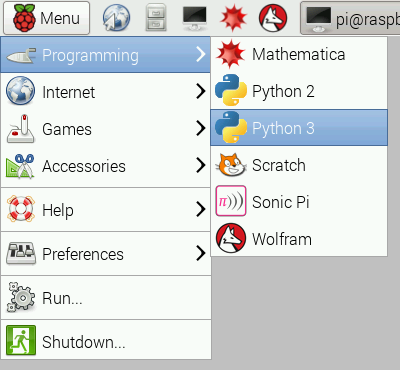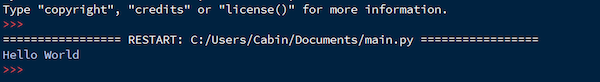In this article, learn how to use the programming language Python to create projects in Raspbian for your Raspberry Pi.

Python is a computer language that has been around for a while but only recently has taken off. While languages such as C and C++ are compiled (i.e. turned directly into computer code), Python is not and is instead interpreted by the Python interpreter. This does mean that Python is slower than the C and C++ languages. However, Python — and most of its libraries — are cross-platform, which means the code written on a Windows machine requires little or no change to run on a Mac OS or Linux.

On the Raspberry Pi, the only library that is not cross-platform for obvious reasons is the RPI.GPIO library which allows your Python programs to access the GPIO. Yes, that’s right! Python can be used to access GPIO and this results in the combination of object orientation and microcontroller capabilities into one platform. In Raspbian, there are a number of Python IDEs installed by default but the simplest one to use is the official Python 3 IDLE. So, use that to write your first Python program!

### How to Load the IDLE Through Raspbian

In order to write a Python program, the first step requires you to load the IDE. To do this, click the Raspberry Icon (this is the equivalent of the start button), navigate to Programming —> Python —> Python 3 IDLE.Accessing Python 3's IDLE (image credit: raspberrypi.org)

When the IDE loads, click File —> New File. In the window that appears (this is the new file), click File —> Save and then call the file whatever you want (for this example, I will call it main.py).

### Print and Execute

The first task to cover is how you can print text to the console window and how to run your Python programs. First write the following line to your file and then save it:

print("Hello World")

To run this program, click Run —> Run Module (F5) or hit the F5 button on your keyboard. When you run the program, the console window should appear and the words “Hello World” should appear.The print function can be used to print strings, variables, and objects that allow you to see a lot of details including unique IDs and addresses. A good first step is to learn how to use “print” to print strings and variables only. So, the next task is to learn about variables.

### Variables in Python

Variables can be thought of as boxes which let you store things inside them. Variables are called variables because their value can be just about anything including strings, numbers, letters, objects, and even binary data. In languages such as C and C++ you have to explicitly state what type a variable is, but in Python you do not.

Variables are given a name that you can use throughout the program but each variable MUST have a unique name (i.e. no two variables have the same name). The best way to see variables in action is with an example.

```        ```x = 10
y = 20
z = x + y
print(z)```
```

Delete all the code in your file from the previous example and copy this code in. Save the file and then run the program. When the program finishes you should see “30” displayed on the console window. When the program starts it created a variable called “x” and then gave it a value of 10. It then created a variable called “y” and gave it the value of 20. Then the program created a variable called “z” and gave it the value of x + y (which is 30). The last instruction the program executed was to print z.

Do take note that sentences have speech marks around them whereas variable names do not.

```        ```print(z)   	# This will print 30
print("z")      	# This will print z```
```

### Basic Input and Flow Control

Programs with predefined variables are a tad boring, but getting user input allows you to make much more complex programs. This is where a very useful function called “input” comes in. Input prompts the user to enter either a number or text. So, like the example before, print the result of two variables added together. However, this time, a user can enter the value of these variables.

```        ```print("Enter value for x: ")
x = int(input())
print("Enter value for y: ")
y = int(input())
z = x + y
print("Value of z=")```
```

When you run the program, the console will ask you for values of x and y. Then it will print the addition of the two. You may have noticed the “int” before the input function; this is needed because, by default, input() returns a string and not a number (i.e. a sentence instead of a value). Because of this, you need to convert that sentence to a numeric value. You can convert just about anything into a numeric value by using the function “int( your value here )”. Below are some examples.

```        ```x = int("10")      	# x = numeric value of the sentence 10
x = int(input())		# x = numeric value of entered text from user```
```

The same applies to converting anything into strings using the function “str(your value here)”.

```        ```x = str(10) 		# x = “10” and not 10
x = str(y)			# x = value of y as a word
# If y = 20 then x = “20”```
```

At this point, the program is still very linear, and cannot make any decisions. This is where flow control statements come in. Flow control statements are essentially responsible for every single program ever made. For now, look at the “IF” statement.

IF statements can compare different values and perform an action depending on whether a comparison is true or not. For example, IF two objects are equal THEN do something. IF NOT, then do something else. Here’s an example:

```        ```print("Enter value for x: ")
x = int(input())
print("Enter value for y: ")
y = int(input())

if(x > y):
print("x is larger than y")
else:
print("y is either smaller or equal to x")```
```

When this program is executed the console will ask for values of x and y. Then it will compare these two values and if x is larger than y then it will print (“x is larger than y”). Otherwise, it will print (“y is either smaller or equal to x”).

One positive aspect of the Python language is its readability (i.e., code is written in a similar fashion to sentences). However, even Python code can be obscure or difficult to follow. It’s at these times that comments come to the rescue!

Comments are pieces of text in your code that are ignored by Python, so you can write whatever you want as a comment. This could include code explanation, what variables do, and how to use a function. Any text after the “#” symbol is considered a comment so text before the “#” on the same line are considered code. You may have noticed the use of the "#" symbol earlier in the article, but below is an example of how code and comments can be on the same line.

```        ```x = 10			# Here we set x to 10
y = x * x			# Now we square x
print(y)			# Tell the user the value of y```
```

Featured image courtesy of Les Pounder